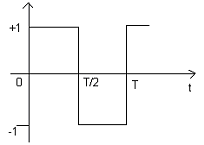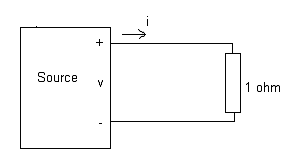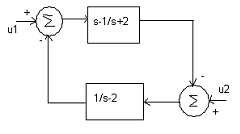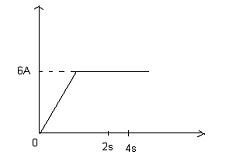# Electrical Engineering test questions for exams and entrance - 1

## Electrical Engineering test questions for exams and entrance - 1

(1) The statement that is not true for a continuous time causal and stable LTI system is

(A) Zeros of the system can lie anywhere in the s-plane
(B) All the poles must lie within | s | = 1.
(C) All the poles of the system must lie on the left side of the j? axis.
(D) All the roots of the characteristic equation must be located on the left side of the jω axis

ANSWER: All the poles must lie within | s | = 1.

(2) Let v = 2x²y + 3y²z + 4z²x. The curl of the gradient of the scalar field v is

(A) 0
(B) ∞
(C) 4ax + 6ay + 8az
(D) None of the above

(3) On which factor the slip of an induction motor normally does not depend?

(A) Shaft torque
(B) Rotor speed
(C) Core loss component
(D) Synchronous speed

(4) For finding mutual inductance the bridge method that is commonly used is

(A) Schering bridge
(B) Heaviside Campbell bridge
(C) Wein bridge
(D) De sauty bridge

(5) Which one of the following statement is true for a 4-point starter? A 4-point starter is used to start and control the speed of a

(A) Dc compound motor
(B) Dc series motor
(C) Dc shunt motor with field weakening control
(D) Dc shunt motor with armature resistance control

ANSWER: Dc shunt motor with armature resistance control

(6) The low-pass filter and high-pass filter are cascaded with each other. Cut-off frequency of low-pass filter and high-pass filter are 30Hz and 20 Hz respectively. The resultant system

of filters will function as

(A) An all-stop filter
(B) An all-pass filter
(C) A band-pass filter
(D) A band-stop filter

(7) For the periodic waveform given below, the second harmonic component has amplitude of(A) 1
(B) 0
(C) 2/π
(D) √5

(8) Consider the figure given below. A source has a load line v + i = 100.The current through the resistance is(A) 150 A
(B) 100 A
(C) 50 A
(D) 25 A

(9) The base load power plants are

(A) Nuclear, run-of-river and diesel
(B) Nuclear and run-of-river
(C) Run-of-river and pump storage
(D) Pump storage and diesel

(10) Consider a transmission line that has a sending end voltage V. For a fixed value of complex power flow in such a transmission line, the real power loss will be proportional to

(A) 1/V²
(B) V
(C) V²
(D) 1/V

(11) Let Q be a (5 x 6) matrix. The rank of Q is 4. The statement, which holds correct for Q, is

(A) Qt Q will be invertible
(B) Q Q t will be invertible
(C) Q will have four linearly independent rows and five linearly independent columns
(D) Q will have four linearly independent rows and four linearly independent columns.

ANSWER: Q will have four linearly independent rows and four linearly independent columns.

(12) Let uniformly distributed random variable be X. X takes the values between 0 and 1. The value of E {X³} will be

(A) 0
(B) 1
(C) 1/2
(D) 1/4

(13) The firing angle of a single-phase fully controlled thyristor bridge ac-dc converter is 25 and an overlap angle is 10. The constant dc output current is 20 A. The fundamental power

factor (displacement factor) at input ac mains is

(A) 0.8
(B) 0.78
(C) 0.9
(D) 1.2

(14) The system shown below is(A) Stable for input u1 but unstable for input u2
(B) Conditionally stable
(C) Unstable
(D) Stable

ANSWER: Stable for input u1 but unstable for input u2

(15) An electrically short, medium and long line primarily depends on the

(A) Power transmitted over the line
(B) Nominal voltage of the line
(C) Physical length of the line
(D) Wavelength of the line

ANSWER: Physical length of the line

(16) Considering the cost and overall effectiveness, the circuit breaker that is best suited for capacitor bank switching is

(A) Air blast
(B) Vacuum
(C) SF6
(D) Oil

(17) For the voltage u (t) = 3 + 4cos(3t), the RMS value is

(A) √17 V
(B) 3 V
(C) 4 V
(D) (3 + 2√2) V

(18) Which one of the following statement holds true for High voltage dc transmission line? HVDC transmission is mainly used for

(A) Minimizing harmonics at the converter stations.
(B) Eliminating reactive power requirement in the operation.
(C) Interconnecting two systems with the same nominal frequency.
(D) Bulk power transmission over very long distances.

ANSWER: Bulk power transmission over very long distances.

(19) Consider a long solenoid of length 1000mm.It is wound uniformly with 3000 turns on a cylindrical paper tube of 60 mm diameter. The inductance of solenoid is

(A) 3.2 μH
(B) 0.32 mH
(C) 32.0 mH
(D) 3.2 mH

(20) _____________ has the highest power factor at full load

(A) Shaded pole type of single-phase induction motor
(B) Capacitor-run type of single-phase induction motor
(C) Capacitor-start type of single-phase induction motor
(D) Split phase type of single-phase induction motor

ANSWER: Capacitor-run type of single-phase induction motor

(21) Figure shows the waveform of the current passing through an inductor. Inductor has resistance of 1 ohm and inductance 2H. The energy absorbed by the inductor in the first four seconds

is(A) 168J
(B) 100J
(C) 98J
(D) 144J

(22) Consider a single-phase transformer. Maximum efficiency of transformer is 90% at full load and unity power factor. What will be the efficiency at half load at same power factor?

(A) 87.8 %
(B) 88.9%
(C) 89.6%
(D) 98.2%

(23) Consider a passive steady state 2-port network. Compared to its input, the steady state output can never offer

(A) Better regulation
(B) Greater power
(C) Lower impedance
(D) Higher voltage

(24) If two incandescent light bulbs of 40 W and 60 W rating are connected in series across mains then

(A) The bulbs together consumes 50 W
(B) The bulbs together consumes 100 W
(C) The 40 W bulb glows brighter
(D) The 60 W bulb glows brighter

ANSWER: The 40 W bulb glows brighter

(25) It is desirable to eliminate 5th harmonic voltage from the phase voltage on an alternator. The coils should be short-pitched by an electrical angle of

(A) 63°
(B) 72°
(C) 30°
(D) 36°